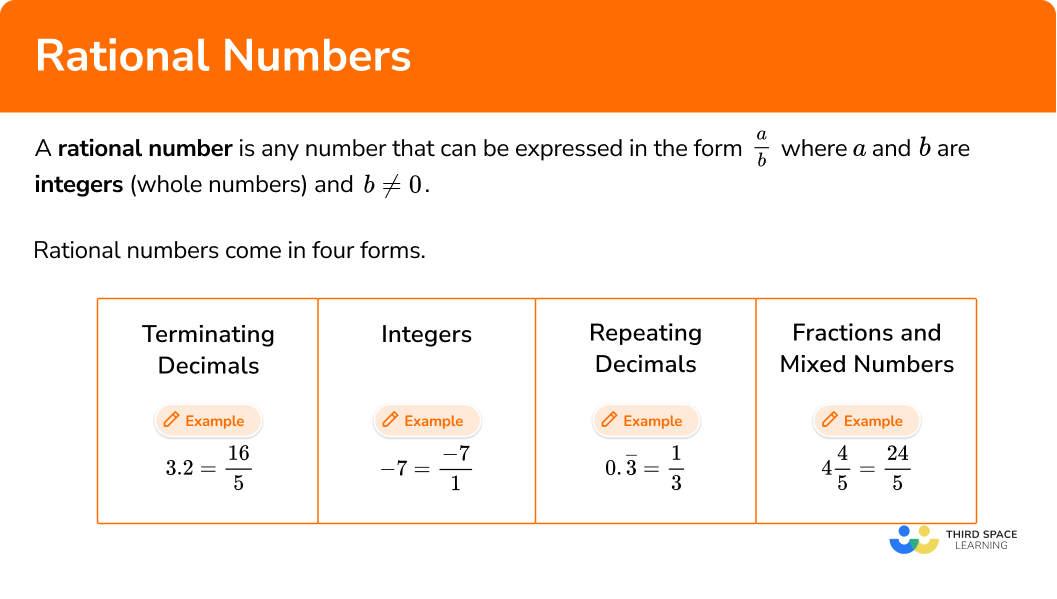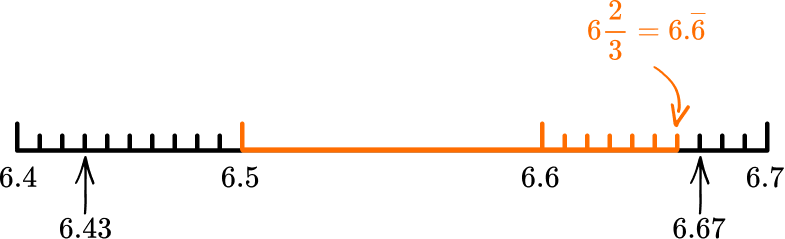# Rational numbers

Here you will learn about rational numbers, including the definition of a rational number, examples of rational numbers and how to identify rational numbers.

Students will first learn about rational numbers as part of the number system in 6th grade.

## What are rational numbers?

Rational numbers are numbers that can be expressed in the form \cfrac{a}{b} where a and b are integers (whole numbers) and b 0. They include natural numbers, whole numbers and integers.

Rational numbers come in four forms. Below are examples of each. Each example has been expressed as a fraction in the form \frac{a}{b} to show that it is rational.

3.2=\cfrac{16}{5}

Terminating decimals
-7=\cfrac{-7}{1}

Integers
0 . \overline{3}=\cfrac{1}{3}

Repeating decimals
4 \cfrac{4}{5} \, =\cfrac{24}{5}

Fractions and mixed numbers

### Key points on rational numbers

• A rational number must have a non-zero denominator

For rational numbers expressed as fractions in the form \cfrac{a}{b}, \; b must be a non-zero integer because zero cannot be a divisor. (Try 5 \div 0 on your calculator and it will give you an error message)

The letter a however can be equal to 0 as you can divide 0 by any real number and get the solution 0. This means that 0 itself is a rational number.

• Numbers that are not rational are called irrational numbers

If a number cannot be represented as a fraction in the form \cfrac{a}{b} where a and b are integers, then the number is irrational.

There are a several famous irrational numbers including

\text { Pi }(\pi=3.141 \ldots) \text {, The Golden Ratio }(\varphi=1.618 \ldots) \text {, and Euler's Number }(e=2.718 \ldots)

• Not all fractions are rational numbers

All rational numbers can be expressed as a fraction, but not all fractions are rational numbers.

For example, \cfrac{5}{\sqrt{2}} is a fraction but it is not rational. The numerator is an integer but the denominator is not (the square root of 2 is irrational). Therefore this fraction does not meet the definition of a rational number.

### What are rational numbers?## Common Core State Standards

How does this relate to 6th grade math?

• Grade 6 – The Number System (6.NS.C.6)
Understand a rational number as a point on the number line. Extend number line diagrams and coordinate axes familiar from previous grades to represent points on the line and in the plane with negative number coordinates.

## How to identify rational numbers

In order to identify and then show that a number is rational:

1. Identify if the number is any of the following. If it is then it is a rational number.
An integer
A terminating decimal
A repeating decimal
A fraction in the form \bf{\cfrac{\textbf{a}}{\textbf{b}}} or a mixed number in the form \bf{C\cfrac{\textbf{a}}{\textbf{b}}} where \textbf{a} and \textbf{b} are
integers
2. Show that the number is rational by writing it as a fraction in the form \bf{\cfrac{\textbf{a}}{\textbf{b}}} where
\textbf{a} and \textbf{b} are integers.

## Rational numbers examples

### Example 1: showing that a terminating decimal is rational

Show that 6.7 is a rational number by expressing it as a fraction in the form \cfrac{a}{b} where a and b are integers.

1. Identify if the number is any of the following. If it is then it is a rational number.
An integer
A terminating decimal
A repeating decimal
A fraction in the form \bf{\cfrac{\textbf{a}}{\textbf{b}}} or a mixed number in the form \bf{C\cfrac{\textbf{a}}{\textbf{b}}} where \textbf{a} and \textbf{b} are
integers

6.7 is a terminating decimal and is therefore rational.

2Show that the number is rational by writing it as a fraction in the form \bf{\cfrac{\textbf{a}}{\textbf{b}}} where
\textbf{a} and \textbf{b} are integers.

6.7 = 6\cfrac{7}{10} = \cfrac{67}{10}

### Example 2: showing that a terminating decimal is rational

Show that 0.045 is a rational number by expressing it as a fraction in the form \cfrac{a}{b} where a and b are integers.

0.045 is a terminating decimal and is therefore rational.

0.045 = \cfrac{45}{1,000} = \cfrac{9}{200}

### Example 3: showing that an integer

Show that -17 is a rational number by expressing it as a fraction in the form \cfrac{a}{b} where a and b are integers.

-17 is an integer and is therefore rational.

-17=\cfrac{-17}{1}

### Example 4: showing that a mixed number is rational

Show that 3\cfrac{4}{5} is a rational number by expressing it as a fraction in the form \cfrac{a}{b} where a and b are integers.

3\cfrac{4}{5} is a mixed number in the form C\cfrac{a}{b} where a, b and C are integers, and is therefore rational.

3\cfrac{4}{5} = \cfrac{3 \; \times \; 5 \; + \; 4}{5} = \cfrac{19}{5}

### Example 5: showing that a repeating decimal is rational

Show that 0 . \overline{6} is a rational number by expressing it as a fraction in the form \cfrac{a}{b} where a and b are integers.

0 . \overline{6} is a repeating decimal and is therefore rational.

0 . \overline{6} = 2 \times 0 . \overline{3} = 2 \times \cfrac{1}{3} = \cfrac{2}{3}

### Example 6: showing that a repeating decimal is rational

Show that 0 . \overline{4} is a rational number by expressing it as a fraction in the form \cfrac{a}{b} where a and b are integers.

0 . \overline{4} is a repeating decimal and is therefore rational.

0 . \overline{4}=4 \times 0 . \overline{1}=4 \times \cfrac{1}{9}=\cfrac{4}{9}

### Teaching tips for rational numbers

• Let students spend time exploring rational numbers using a calculator. This gives them an opportunity to notice patterns and also easily convert between fractions and decimals.

• Have students create a number line of their life with rational numbers – their birth starting at 0. They should plot things that happened before their birth with negative rational numbers and things that happened after their birth with positive rational numbers. Challenge them to represent each rational number on their number line in as many ways as possible.

• Just as developing whole number sense takes time and a variety of experiences in elementary school, so does understanding of rational numbers in middle school. Fit in quick reviews to rational numbers throughout the year or make connections as they appear in other standards to help deepen student understanding over time.

### Our favorite mistakes

• Thinking zero is not a rational number
Zero is a rational number because it can be written as \cfrac{0}{n} for any value of n other than 0.

• Thinking that all fractions are rational numbers
All rational numbers can be written as fractions but not all fractions are rational numbers. If the fraction is in the form \cfrac{a}{b} and a and b are integers and b 0 then the number is rational. However if a or b are not integers then the fraction could represent an irrational number.
E.g.
\cfrac{\sqrt{2}}{3} is a fraction which is irrational.

• Non-terminating decimals and rational numbers
Some non-terminating decimals are irrational and others are rational.
E.g.
\sqrt{3} = 1.73205… \; This is a non terminating irrational number.
\cfrac{7}{9} = 0.777… \; This is a non terminating rational number.

### Practice rational numbers questions

1. Which of the following numbers is not rational?

\cfrac{2}{7}\sqrt{27}70.2 \overline{7}• \cfrac{2}{7} is a fraction in the form \cfrac{a}{b} where a and b are integers
• 0.2 \overline{7} is a repeating decimal.
• 7 is an integer

All of these are types of rational numbers.

\sqrt{27} = 5.196152… This is non terminating decimal which is not repeating and it cannot be expressed as a fraction in the form \cfrac{a}{b} where a and b are integers. It is therefore irrational.

2. Show that -4 is a rational number by expressing it as a fraction in the form \cfrac{p}{q} where p and q are integers.

\cfrac{-4}{4}\cfrac{1}{-4}\cfrac{-4}{1}\cfrac{4}{-4}Any integer, like -4, can be shown as a fraction by placing it over 1.

-4=\cfrac{-4}{1}

3. Why is 0 . \overline{7} a rational number?

Because it is a repeating decimalBecause it is an integerBecause it is a terminating decimalBecause it is a mixed number0 . \overline{7} is a non-terminating decimal and a repeating decimal.

Not all non-terminating decimals are rational, but all repeating decimals are rational because they can be expressed as fractions in the form \cfrac{a}{b} where a and b are integers.

0 . \overline{7}=7 \times \cfrac{1}{9}=\cfrac{7}{9}

4. Show that 3.5 is a rational number by expressing it as a fraction in the form \cfrac{p}{q} where p and q are integers.

\cfrac{3}{5}\cfrac{5}{3}\cfrac{7}{2}\cfrac{2}{7}3.5 = 3\cfrac{5}{10} = 3\cfrac{1}{2} = \cfrac{3 \; \times \; 2 \; + \; 1}{2} = \cfrac{7}{2}

5. Show that 0 . \overline{8} is a rational number by expressing it as a fraction in the form \cfrac{a}{b} where a and b are integers.

\cfrac{0}{8}\cfrac{0.\overline{8}}{10}\cfrac{8}{9}\cfrac{4}{5}0.\overline{8} = 8 \times 0.\overline{1} = 8 \times \cfrac{1}{9} = \cfrac{8}{9}

6. Which one of these numbers is a rational number that lies between 6.5 and 6\cfrac{2}{3}?

6.76.436.676.6A number between 6.5 and 6 \cfrac{2}{3} (6 . \overline{6}=6.6666) would fall here on the number line:6.67 and 6.7 are rational, but too big.
6.23 is rational, but too small.

6.6 is rational and is in between.

## Rational numbers FAQs

Can rational numbers be expressed in decimal form?

Yes, rational numbers can be shown as terminating or repeating decimals.

How many rational numbers are there?

There are an infinite number of rational numbers within our number system. In fact, even between any two given numbers there are an infinite number of rational numbers.

Are there other types of numbers besides rational?

Yes, there are irrational numbers, which students learn about later in middle school. In high school students also learn about real numbers, imaginary numbers and complex numbers.

## Still stuck?

At Third Space Learning, we specialize in helping teachers and school leaders to provide personalized math support for more of their students through high-quality, online one-on-one math tutoring delivered by subject experts.

Each week, our tutors support thousands of students who are at risk of not meeting their grade-level expectations, and help accelerate their progress and boost their confidence.